# 2w字 | 28道 LeetCode 题目带你看看链表的那些套路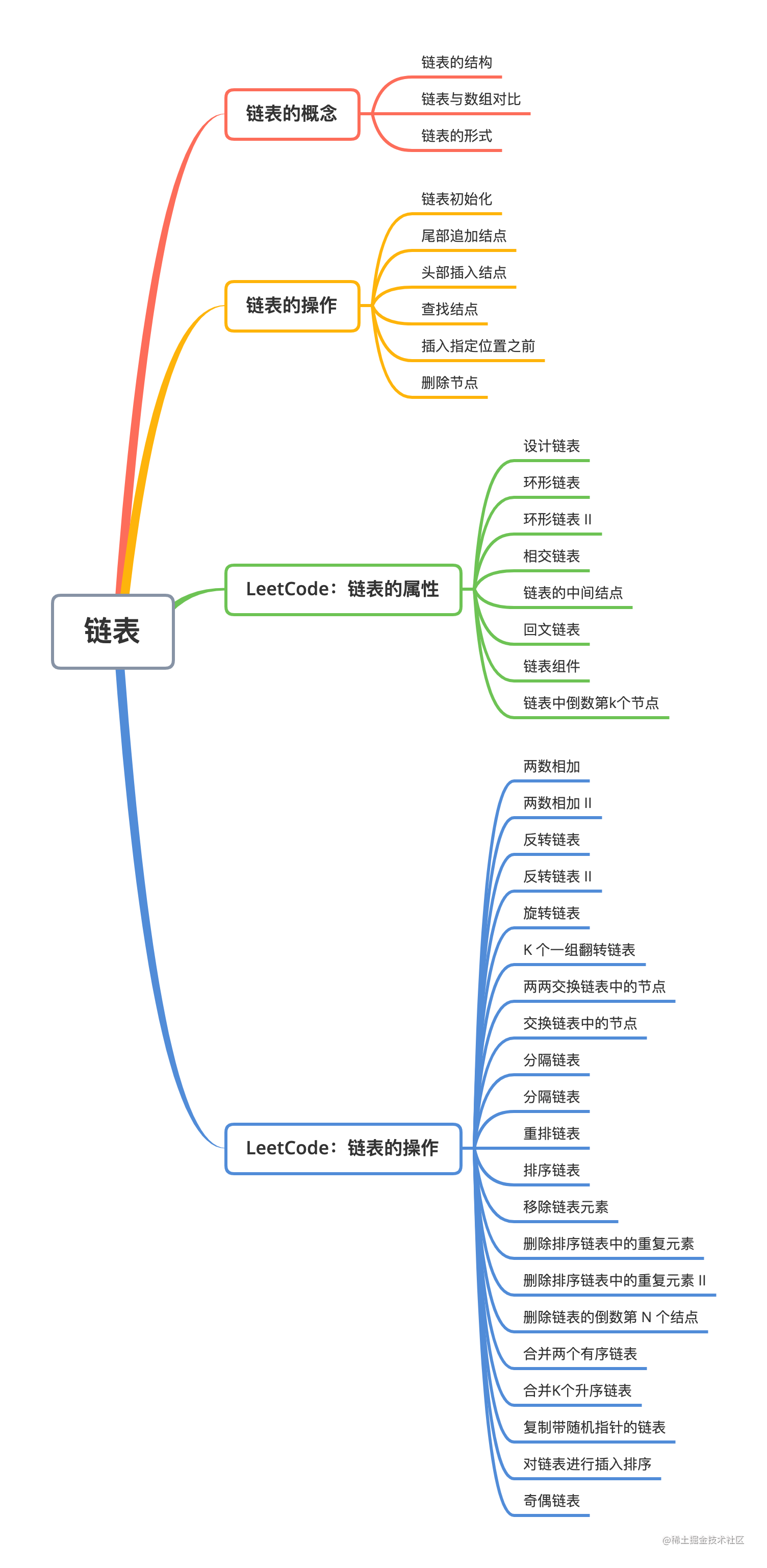### 1. 链表的概念

#### （1）链表的结构

``````function ListNode(val) {
this.val = val;
this.next = null;
}

#### （2）链表与数组对比

1）空间利用率 数组在创建之后大小是无法改变的，想要增加元素的话就必须重新创建一个新的数组。所，以有时为了能够动态地增加元素，在开始创建数组时会声明一个比需要的大小还多的空间出来，以便后面添加新的元素。这个时候就会造成空间上的浪费，所以，数组的空间利用率相当于本来需要的大小除以创建出来数组的大小。

2）时间复杂度 访问数组元素的时间复杂度是 O(1)。而因为链表顺序访问的这个特性，访问链表中第 N 个元素需要从第一个元素一直遍历到第 N 个元素，所以平均下来的时间复杂度是 O(N)。

#### （3）链表的形式

1）单向链表 所有的链表节点中都只保存了指向下一个节点地址的信息。这种在一个节点中既保存了数据，也保存了指向下一个节点地址信息的链表，称之为单向链表（Singly Linked List）。如下图所示：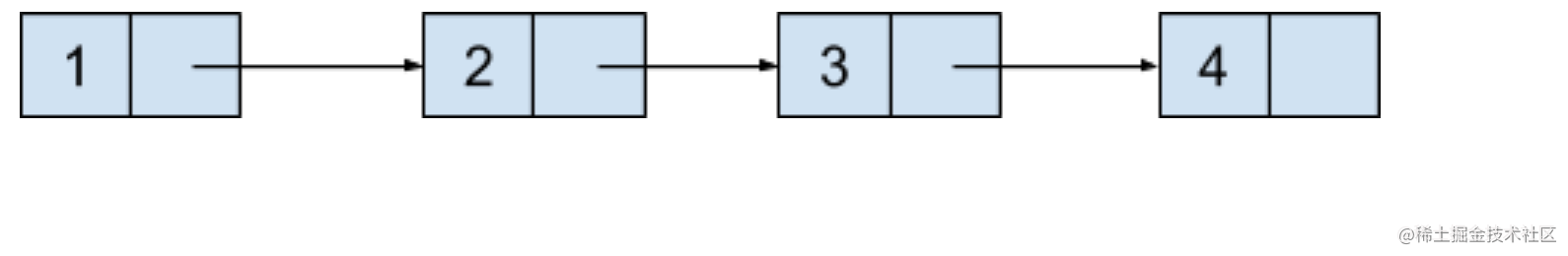2）双向链表 单向链表有着只能朝着一个方向遍历的局限性，既然可以保存指向下一个节点地址的信息，也可以保存指向上一个节点地址的信息。这种在一个节点中保存了数据也保存了连向下一个和上一个节点地址信息的链表，称之为双向链表（Doubly Linked List）。和链表中尾节点的下一个节点只保存空地址一样，链表中头节点的上一个节点地址也保存着空地址，如下图所示：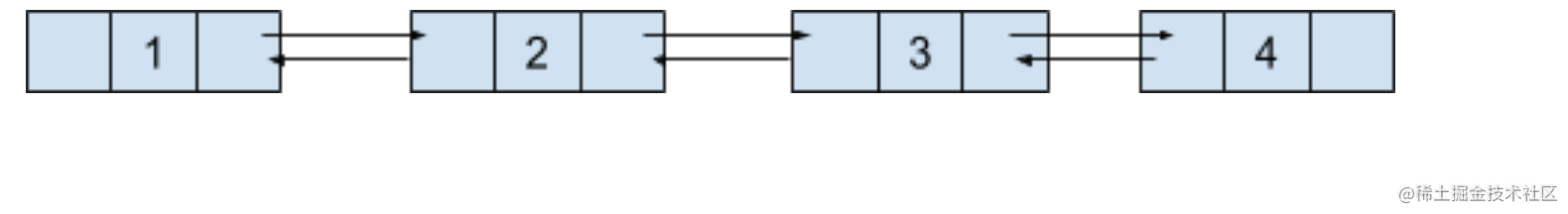（3）循环链表 无论是单向链表或者是双向链表，当遍历至尾节点之后就无法再遍历下去了，如果将尾节点指向下一个节点地址的信息更新成指向头节点的话，这样整个链表就形成了一个环，这种链表称之为循环链表（Circular Linked List）。如下图所示：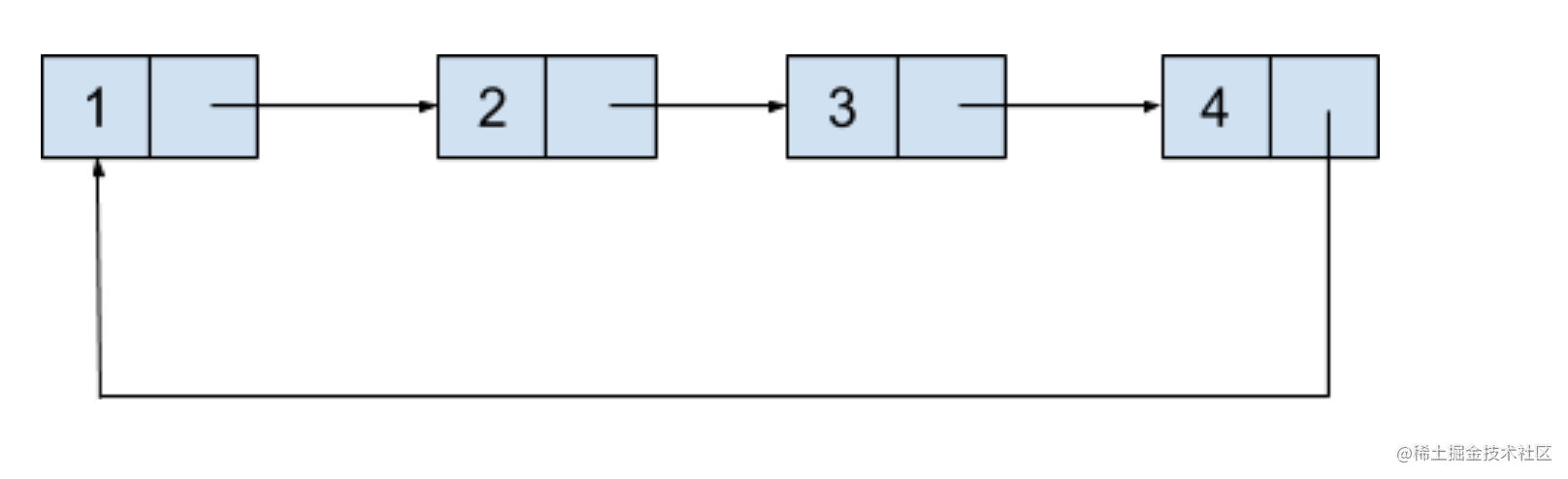### 2. 链表的操作

• 链表初始化
• 尾部追加结点
• 头部插入结点
• 查找结点
• 插入指定位置之前
• 删除结点

``````var MyLinkedList = function() {
/* code here: 初始化链表 */
};

/* code here: 将值为 val 的结点追加到链表尾部 */
};

/* code here: 插入值val的新结点，使它成为链表的第一个结点 */
};

/* code here: 获取链表中第index个结点的值。如果索引无效，则返回-1 */
// index从0开始。
};

// code here:
// 在链表中的第 index 个结点之前添加值为 val  的结点。
// 1. 如果 index 等于链表的长度，则该结点将附加到链表的末尾。
// 2. 如果 index 大于链表长度，则不会插入结点。
// 3. 如果 index 小于0，则在头节点前插入
};

/* code here: 如果索引index有效，则删除链表中的第index个结点 */
};

#### （1）链表初始化

``````var listNode = function(val) {
this.val = val
this.next = null
};

this.dummy = new listNode()
this.tail = this.dummy
this.length = 0
};

• 动：tail 指针在链表发生变动的时候，就需要移动调整。

#### （2）尾部追加结点

``````MyLinkedList.prototype.addAtTail = function(val) {
// 尾部添加一个新结点
this.tail.next = new listNode(val)
// 移动tail指针
this.tail = this.tail.next;
// 链表长度+1
this.length ++
};

#### （3）头部插入结点

• 新结点 p.next 指向 dummy.next；
• dummy.next 指向 p；
• 如果原来的 tail 指向 dummy，那么将 tail 指向 p。

``````MyLinkedList.prototype.addAtHead = function(val) {
// 生成一个结点，存放的值为val
const p = new listNode(val)
// 将p.next指向第一个结点
p.next = this.dummy.next;
// dummy.next指向新结点，使之变成第一个结点
this.dummy.next = p;
// 注意动静结合原则，添加结点时，注意修改tail指针。
if (this.tail == this.dummy) {
this.tail = p;
}
// 链表长度+1
this.length ++
};

#### （4）查找结点

• 通过 prev.next 就可以访问到想要找到的结点，如果没有找到，那么 prev.next 为 null；
• 通过 prev 可以方便完成后续操作，比如在 target 前面 insert 一个新结点，或者将 target 结点从链表中移出去。

``````MyLinkedList.prototype.getPreNode = function(index) {
if (index < 0 || index >= this.length) {
return -1;
}
// 初始化front与back，分别一前一后
let front = this.dummy.next
let back = this.dummy
// 在查找的时候，front与back总是一起走
for (let i = 0; i < index && front != null; i++) {
back = front;
front = front.next;
}
// 把back做为prev并且返回
return back
};

• 如果 target 在链表中不存在，此时 prev 返回链表的最后一个结点；
• 如果为空链表（空链表指只有一个假头的链表），此时 prev 指向 dummy。也就是说，返回的 prev 指针总是有效的。

``````MyLinkedList.prototype.get = function(index) {
// 获取链表中第 index 个结点的值。如果索引无效，则返回-1。
// index从0开始
if (index < 0 || index >= this.length) {
return -1;
}
// 因为getPrevNode总是返回有效的结点，所以可以直接取值。
return this.getPreNode(index).next.val
};

#### （5）插入指定位置之前

• 如果 index 大于链表长度，则不会插入结点。
• 如果 index 等于链表的长度，则该结点将附加到链表的末尾。
• 如果 index 小于 0，则在头部插入结点。
• 否则在指定位置前面插入结点。

• 使用 getPrevNode() 函数拿到 index 之前的结点 pre；
• 在 pre 的后面添加一个新结点。

``````MyLinkedList.prototype.addAtIndex = function(index, val) {
if (index > this.length) {
// Case 1.如果 index 大于链表长度，则不会插入结点。
return;
} else if (index == this.length) {
// Case 2.如果 index 等于链表的长度，则该结点将附加到链表的末尾。
} else if (index <= 0) {
// Case 3. 如果index小于0，则在头部插入结点。
} else {
// Case 4.
// 得到index之前的结点pre
const pre = this.getPreNode(index);
// 在pre的后面添加新结点
const p = new listNode(val);
p.next = pre.next;
pre.next = p;
// 链表长度+1
this.length++;
}
};

#### （6）删除节点

• 如果 index 无效，那么什么也不做；
• 如果 index 有效，那么将这个结点删除。

``````MyLinkedList.prototype.deleteAtIndex = function(index) {
// Case 1. 如果index无效，那么什么也不做。
if (index < 0 || index >= this.length) {
return;
}
// Case 2. 删除index结点
// step 1. 找到index前面的结点
const pre = this.getPreNode(index);
// step 2. 如果要删除的是最后一个结点，那么需要更改tail指针
if (this.tail == pre.next) {
this.tail = pre;
}
// step. 3 进行删除操作。并修改链表长度。
pre.next = pre.next.next;
this.length--;
};

#### （7）总结

``````        /**
* Initialize your data structure here.
*/
var listNode = function(val) {
this.val = val
this.next = null
};

this.dummy = new listNode()
this.tail = this.dummy
this.length = 0
};

/**
* Get the value of the index-th node in the linked list. If the index is invalid, return -1.
* @param {number} index
* @return {number}
*/
if (index < 0 || index >= this.length) {
return -1;
}
// 初始化front与back，分别一前一后
let front = this.dummy.next
let back = this.dummy
// 在查找的时候，front与back总是一起走
for (let i = 0; i < index && front != null; i++) {
back = front;
front = front.next;
}
// 把back做为prev并且返回
return back
};

if (index < 0 || index >= this.length) {
return -1;
}

return this.getPreNode(index).next.val
};

/**
* Add a node of value val before the first element of the linked list. After the insertion, the new node will be the first node of the linked list.
* @param {number} val
* @return {void}
*/
// 生成一个结点，存放的值为val
const p = new listNode(val)
// 将p.next指向第一个结点
p.next = this.dummy.next;
// dummy.next指向新结点，使之变成第一个结点
this.dummy.next = p;
// 注意动静结合原则，添加结点时，注意修改tail指针。
if (this.tail == this.dummy) {
this.tail = p;
}
// 链表长度+1
this.length ++
};

/**
* Append a node of value val to the last element of the linked list.
* @param {number} val
* @return {void}
*/
// 尾部添加一个新结点
this.tail.next = new listNode(val)
// 移动tail指针
this.tail = this.tail.next;
// 链表长度+1
this.length ++
};

/**
* Add a node of value val before the index-th node in the linked list. If index equals to the length of linked list, the node will be appended to the end of linked list. If index is greater than the length, the node will not be inserted.
* @param {number} index
* @param {number} val
* @return {void}
*/
if (index > this.length) {
// Case 1.如果 index 大于链表长度，则不会插入结点。
return;
} else if (index == this.length) {
// Case 2.如果 index 等于链表的长度，则该结点将附加到链表的末尾。
} else if (index <= 0) {
// Case 3. 如果index小于0，则在头部插入结点。
} else {
// Case 4.
// 得到index之前的结点pre
const pre = this.getPreNode(index);
// 在pre的后面添加新结点
const p = new listNode(val);
p.next = pre.next;
pre.next = p;
// 链表长度+1
this.length++;
}
};

/**
* Delete the index-th node in the linked list, if the index is valid.
* @param {number} index
* @return {void}
*/

// Case 1. 如果index无效，那么什么也不做。
if (index < 0 || index >= this.length) {
return;
}
// Case 2. 删除index结点
// step 1. 找到index前面的结点
const pre = this.getPreNode(index);
// step 2. 如果要删除的是最后一个结点，那么需要更改tail指针
if (this.tail == pre.next) {
this.tail = pre;
}
// step. 3 进行删除操作。并修改链表长度。
pre.next = pre.next.next;
this.length--;
};

/**
* var obj = new MyLinkedList()
* var param_1 = obj.get(index)
* obj.deleteAtIndex(index)
*/

``````/**
* Initialize your data structure here.
*/
this.rear=null
this.len=0
};
function ListNode(val) {
this.val = val;
this.next = null;
}
/**
* Get the value of the index-th node in the linked list. If the index is invalid, return -1.
* @param {number} index
* @return {number}
*/
if(index<0||index>this.len-1)
return -1
while(index-->0){
if(node.next==null)
return -1
node=node.next
}
return node.val
};

/**
* Add a node of value val before the first element of the linked list. After the insertion, the new node will be the first node of the linked list.
* @param {number} val
* @return {void}
*/
var node=new ListNode(val)
this.rear=node
else
this.len++
};

/**
* Append a node of value val to the last element of the linked list.
* @param {number} val
* @return {void}
*/
var node=new ListNode(val)
else
this.rear.next=node
this.rear=node
this.len++
};

/**
* Add a node of value val before the index-th node in the linked list. If index equals to the length of linked list, the node will be appended to the end of linked list. If index is greater than the length, the node will not be inserted.
* @param {number} index
* @param {number} val
* @return {void}
*/
if(index<=0)
if(this.len<index)
return
if(index==this.len)
while(index-->1){
node=node.next
}

var newnode=new ListNode(val)
newnode.next=node.next
node.next=newnode
this.len++
};

/**
* Delete the index-th node in the linked list, if the index is valid.
* @param {number} index
* @return {void}
*/
if(index<0||index>this.len-1||this.len==0)
return
if(index==0){
this.len--
return
}

var myindex=index
while(index-->1){
node=node.next
}
if(myindex==(this.len-1)){
this.rear=node
}
node.next=node.next.next
this.len--
};

/**
* var obj = new MyLinkedList()
* var param_1 = obj.get(index)
* obj.deleteAtIndex(index)
*/

### 3. 经典题目：链表的属性

#### （1）环形链表

``````输入：head = [3,2,0,-4], pos = 1

``````输入：head = [1,2], pos = 0

``````输入：head = , pos = -1

``````/**
* function ListNode(val) {
*     this.val = val;
*     this.next = null;
* }
*/

/**
* @return {boolean}
*/
return true
}else{
}
}
return false
};

• 时间复杂度：O(n)，其中n是链表的节点数，最差坏的情况下我们要遍历完整个链表；
• 空间复杂度：O(n)，其中n是链表的节点数，主要为哈希表的开销，最坏情况下需要将每个节点插入到哈希表中一次。

#### （2）环形链表 II

``````输入：head = [3,2,0,-4], pos = 1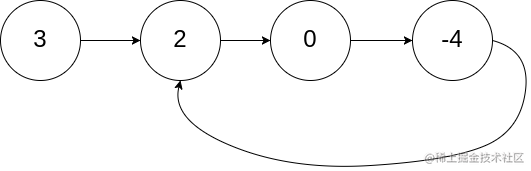``````输入：head = [1,2], pos = 0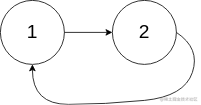``````输入：head = , pos = -1``````/**
* function ListNode(val) {
*     this.val = val;
*     this.next = null;
* }
*/

/**
* @return {ListNode}
*/
}else{
}
}
return null
};

``````/**
* function ListNode(val) {
*     this.val = val;
*     this.next = null;
* }
*/

/**
* @return {ListNode}
*/

while(fast && fast.next && fast.next.next){
slow = slow.next
fast = fast.next.next
if(fast == slow){
while(cur!=slow){
cur = cur.next
slow = slow.next
}
return slow
}
}
return null
};

• 时间复杂度：O(n)，其中n是链表的节点数，最差坏的情况下我们要遍历完整个链表；
• 空间复杂度：O(n)，其中n是链表的节点数，主要为哈希表的开销，最坏情况下需要将每个节点插入到哈希表中一次。

• 时间复杂度：O(n)，其中n是链表中节点数。在最初判断快慢指针是否相遇时，slow 指针走过的距离不会超过链表的总长度；随后寻找入环点时，走过的距离也不会超过链表的总长度。因此，总的执行时间为 O(N)+O(N)=O(N)。
• 空间复杂度：O(1)。我们只使用了 slow、fast、cur 三个指针。

#### （3）相交链表

• 如果两个链表没有交点，返回 null.
• 在返回结果后，两个链表仍须保持原有的结构。
• 可假定整个链表结构中没有循环。
• 程序尽量满足 O(n) 时间复杂度，且仅用 O(1) 内存。

• 定义两个指针 pA 和 pB；
• pA 从链表 a 的头部开始走，走完后再从链表 b 的头部开始走；
• pB 从链表 b 的头部开始走，走完后再从链表 a 的头部开始走；
• 如果存在相交的点就直接返回pA或者pB
``````/**
* function ListNode(val) {
*     this.val = val;
*     this.next = null;
* }
*/

/**
* @return {ListNode}
*/
while(pA !== pB){
pA = pA === null ? headB : pA.next
pB = pB === null ? headA : pB.next
}
return pA
};

• 时间复杂度：O(m + n) ，其中m和n分别是两个链表的节点数，最差情况下需要遍历完两个链表。
• 空间复杂度：O(1)，节点指针 A , B 使用常数大小的额外空间。

#### （4）链表的中间结点

``````输入：[1,2,3,4,5]

ans.val = 3, ans.next.val = 4, ans.next.next.val = 5, 以及 ans.next.next.next = NULL.

``````输入：[1,2,3,4,5,6]

``````/**
* function ListNode(val) {
*     this.val = val;
*     this.next = null;
* }
*/
/**
* @return {ListNode}
*/

while(fast && fast.next){
slow = slow.next
fast = fast.next.next
}
return slow
};

• 时间复杂度：O(n)，其中 n 是给定链表的结点数目。
• 空间复杂度：O(1)，只需要常数空间来存放 `slow``fast` 两个指针。

#### （5）回文链表

``````输入: 1->2

``````输入: 1->2->2->1

1）字符串拼接： 对于这道题，最直接的思路就是，遍历链表，同时正向和反向拼接链表的节点，最后比较两个拼接出来的字符串是否一样。**

2）递归遍历：

• 然后，调用递归函数，对链表进行逆序遍历，当头部节点为null的时候停止遍历
• 如果正序遍历的节点值和逆序遍历的节点值都相等，就返回true，否则就返回false

1）字符串拼接：

``````/**
* function ListNode(val) {
*     this.val = val;
*     this.next = null;
* }
*/
/**
* @return {boolean}
*/
let a = ""
let b = ""
a = a + nodeVal
b = nodeVal + b
}
return a === b
};

``````/**
* function ListNode(val) {
*     this.val = val;
*     this.next = null;
* }
*/
/**
* @return {boolean}
*/

let pointer

pointer = pointer.next
return res
}

};

• 时间复杂度：O(n)，其中 n 指的是链表的元素个数，我们需要遍历完整个链表。
• 空间复杂度：O(1)，这里只需要常量的空间来保存两个拼接的字符串。

• 时间复杂度：O(n)，其中 n 指的是链表的大小。
• 空间复杂度：O(n)，其中 n 指的是链表的大小，最差的情况下递归栈的深度为n。

#### （6）链表组件

``````输入:
G = [0, 1, 3]

``````输入:
G = [0, 3, 1, 4]

• 如果 `N` 是给定链表 `head` 的长度，`1 <= N <= 10000`
• 链表中每个结点的值所在范围为 `[0, N - 1]`
• `1 <= G.length <= 10000`
• `G` 是链表中所有结点的值的一个子集.

• 组件必须是链表中的连续节点
• 在G中包含连续节点的每个数值，如果出现不连续，结果就+1

``````/**
* function ListNode(val) {
*     this.val = val;
*     this.next = null;
* }
*/
/**
* @param {number[]} G
* @return {number}
*/
var numComponents = function(head, G) {
let isComponent = false
let res = 0

while(cur){
if(G.indexOf(cur.val) > -1){
if(!isComponent){
isComponent = true
res++
}
}else{
isComponent = false
}
cur = cur.next
}
return res
};

• 时间复杂度：O(m+n)，这里需要遍历完整个整个链表和数组，所以时间复杂度为O(m+n)，其中m是链表的元素个数，n是G的元素个数；
• 空间复杂度：O(1)。需要额外的常数大小的辅助空间。

#### （7）链表中倒数第k个节点

``````给定一个链表: 1->2->3->4->5, 和 k = 2.

``````/**
* function ListNode(val) {
*     this.val = val;
*     this.next = null;
* }
*/
/**
* @param {number} k
* @return {ListNode}
*/
var getKthFromEnd = function(head, k) {
let cur = 0;
while(cur < k){
fast = fast.next
cur++
}

while(fast){
fast = fast.next
slow = slow.next
}
return slow

};

• 时间复杂度： O(n)，其中n是链表的节点数，我们需要遍历整个链表；
• 空间复杂度： O(n)，其中n是链表的节点数，我们需要储存快慢两个指针指向的链表；

### 4. 经典题目：链表的操作

#### （1）两数相加

``````输入：(2 -> 4 -> 3) + (5 -> 6 -> 4)

``````/**
* function ListNode(val) {
*     this.val = val;
*     this.next = null;
* }
*/
/**
* @param {ListNode} l1
* @param {ListNode} l2
* @return {ListNode}
*/
var addTwoNumbers = function(l1, l2) {
const l3 = new ListNode(0)  // 用来保存结果的链表
let p1 = l1 //定义两个指针，来遍历链表
let p2 = l2
let p3 = l3 //定义两个指针，指向结果链表
let carry = 0  // 记录每次相加之后的进位的值
while(p1 || p2){
const v1 = p1 ? p1.val : 0
const v2 = p2 ? p2.val : 0
const val = v1 + v2 + carry //
carry = Math.floor(val/10)  // 计算进位的值
p3.next = new ListNode(val % 10) // 将个位的值作为当前位的结果
if(p1) p1 = p1.next   // 将指针指向下一个节点
if(p2) p2 = p2.next
p3 = p3.next
}
// 如果最后还有进位的值，直接放在结果链表
if(carry){
p3.next = new ListNode(carry)
}
return l3.next
};

• 时间复杂度：O(max(m, n))，其中 m 与 n 分别为两个链表的长度，我们需要遍历每个链表。
• 空间复杂度：O(1)。需要额外的常数大小的辅助空间。

#### （2）两数相加 II

``````输入：(7 -> 2 -> 4 -> 3) + (5 -> 6 -> 4)

• 初始化stack1和stack2两个栈，并遍历两个链表，将链表元素存储起来；
• 当两个栈都为空的时候，结束遍历
• 最后判断一下，如果最后一次相加之后还有进位值，记得加在链表上
``````/**
* function ListNode(val) {
*     this.val = val;
*     this.next = null;
* }
*/
/**
* @param {ListNode} l1
* @param {ListNode} l2
* @return {ListNode}
*/
var addTwoNumbers = function(l1, l2) {
let stack1 = [], stack2 = [];

while(l1){
stack1.push(l1)
l1 = l1.next
}
while(l2){
stack2.push(l2)
l2 = l2.next
}

let carry = 0

while(stack1.length || stack2.length){
let p1 = stack1.pop()
let p2 = stack2.pop()

let x = p1 ? p1.val : 0
let y = p2 ? p2.val : 0

let sum = x + y + carry
let m = sum % 10   //个位
let n = Math.floor(sum / 10)
carry = n
}

if(carry){
}
};

• 时间复杂度：O(max(m, n))，其中 m 与 n 分别为两个链表的长度，我们需要遍历每个链表。
• 空间复杂度：O(m + n)，其中 m 与 n 分别为两个链表的长度，这是把链表内容放入栈中所用的空间。

#### （3）反转链表

``````输入: 1->2->3->4->5->NULL

``````/**
* function ListNode(val) {
*     this.val = val;
*     this.next = null;
* }
*/
/**
* @return {ListNode}
*/
// 设置指针指向前驱节点和当前节点
let pre = null
// 遍历链表，直到链表节点为空
while (cur!= null){
// 记录当前的节点，用于后面的遍历
let next = cur.next
// 调转链表的指针方向
cur.next = pre
// 向后移动指针
pre = cur
cur = next
}
return pre
};

``````/**
* function ListNode(val) {
*     this.val = val;
*     this.next = null;
* }
*/
/**
* @return {ListNode}
*/
// 首先判断链表为空或者只有一个节点的情况
}
// 储存下一个节点，便于后面进行递归
// 递归
let result = reverseList(next)
// 断开当前节点和它的后驱节点
// 把后面的节点作为当前的节点
return result
};

• 时间复杂度：O(n)，其中n是链表的节点数，我们需要遍历整个链表；
• 空间复杂度：O(1)，这里我们只需要常量空间来储存指针。

• 时间复杂度：O(n)，其中n是链表的节点数；
• 空间复杂度：O(n)，其中n是链表的节点数，在最差的情况下递归栈的深度为n。

#### （4）反转链表 II

``````输入: 1->2->3->4->5->NULL, m = 2, n = 4

``````/**
* function ListNode(val) {
*     this.val = val;
*     this.next = null;
* }
*/
/**
* @param {number} m
* @param {number} n
* @return {ListNode}
*/
var reverseBetween = function(head, m, n) {
// 定义虚拟头结点
let dummy = new ListNode()
// 寻找第m-1节点
let p = dummy
for(let i =0; i<m-1; i++){
p= p.next
}
// 定义当前节点和前驱节点，当前节点指向m节点
let pre = null
let cur = p.next
// 将m到n的节点进行反转
for(let i = 0; i<= n-m; i++){
let next = cur.next
cur.next = pre
pre = cur
cur = next
}
// 将反转的局部链表和原链表进行拼接
p.next.next = cur
p.next = pre

return dummy.next
};

• 时间复杂度：O(n)，其中 n 是链表的节点数。在最坏情况下，需要遍历整个链表。

• 空间复杂度：O(1)。需要额外的常数大小的辅助空间。

#### （5）旋转链表

``````输入: 1->2->3->4->5->NULL, k = 2

``````输入: 0->1->2->NULL, k = 4

• 首先遍历链表，找到链表的尾结点，将尾结点和头结点连起来，这样就形成了一个环形链表；
• 题目说的右移k步，这里我们让尾结点左移，相当于往左移动length-k步；
• 最后，移动完成之后，找到链表的尾结点，将环形链表断开为单向链表。
``````/**
* function ListNode(val, next) {
*     this.val = (val===undefined ? 0 : val)
*     this.next = (next===undefined ? null : next)
* }
*/
/**
* @param {number} k
* @return {ListNode}
*/
var rotateRight = function(head, k) {
}
// 找到尾结点，将单链表形成环形链表
let length = 1
while(tail.next){
length++
tail = tail.next
}
// 尾结点进行移动
k = k % length
for(let i = 0; i < length - k; i++){
tail = tail.next
}
// 找到头结点，断开环形链表
tail.next = null
};

• 时间复杂度：O(n)，其中 n 是链表中节点的个数；
• 空间复杂度：O(1)。需要额外的常数大小的辅助空间。

#### （6）K 个一组翻转链表

``````给你这个链表：1->2->3->4->5

• 你的算法只能使用常数的额外空间。
• 你不能只是单纯的改变节点内部的值，而是需要实际进行节点交换。

• 启用一个计数器，截出链表的前k个节点
• 将链表进行反转
• 将上一次遍历时最后的节点，作为当前的开始节点，进行递归操作
``````/**
* function ListNode(val) {
*     this.val = val;
*     this.next = null;
* }
*/
/**
* @param {number} k
* @return {ListNode}
*/
var reverseKGroup = function(head, k) {
let count = 0

while(cur !== null && count !== k){
cur = cur.next
count++
}

if(count == k){
cur = reverseKGroup(cur, k)
// 翻转链表
while(count !== 0){
count--
}
}
};

• 时间复杂度：O(n)，其中 n 是链表中节点的个数；
• 空间复杂度：O(1)。需要额外的常数大小的辅助空间。

#### （7）两两交换链表中的节点

``````输入：head = [1,2,3,4]

``````输入：head = []

``````输入：head = 

• 链表中节点的数目在范围 [0, 100] 内
• 0 <= Node.val <= 100

（1）递归实现 在递归的过程中，其终止条件就是链表中没有节点了，或者链表中只有一个节点，不能再进行交换了。

（2）迭代实现 对于迭代的方式，我们可以创建一个哑结点list，将这个节点指向题目给出的节点的头结点。初始化一个temp，用来保存这个哑结点，初始值为list，每次交换都交换temp后面的两个节点。 只有当temp节点之后有至少两个节点时，才能接续往后进行交换，否则结束交换。交换时，获取temp后面两个节点，更新节点的指针关系，来实现两个节点的交换。当两个节点交换完成之后，记得要更新temp节点为两两交换之后的第二个节点，即node1。

``````/**
* function ListNode(val) {
*     this.val = val;
*     this.next = null;
* }
*/
/**
* @return {ListNode}
*/
}
};

``````/**
* function ListNode(val) {
*     this.val = val;
*     this.next = null;
* }
*/
/**
* @return {ListNode}
*/
}
const list = new ListNode(0)

let temp = list

while(temp.next && temp.next.next){
const node1 = temp.next
const node2 = temp.next.next
// node1 和 node2 进行交换
temp.next = node2
node1.next = node2.next
node2.next = node1
// 更新temp节点
temp = node1
}
return list.next
};

• 时间复杂度：O(n)，其中 n 是链表的节点数量。需要对每个节点进行更新指针的操作。
• 空间复杂度：O(n)，其中 n 是链表的节点数量。空间复杂度主要取决于递归调用的栈空间。

• 时间复杂度：O(n)，其中 n 是链表的节点数量。需要对每个节点进行更新指针的操作。
• 空间复杂度：O(1)。需要额外的常数大小的辅助空间。

#### （8）交换链表中的节点

``````输入：head = [1,2,3,4,5], k = 2

``````输入：head = [7,9,6,6,7,8,3,0,9,5], k = 5

``````输入：head = , k = 1

``````输入：head = [1,2], k = 1

``````输入：head = [1,2,3], k = 2

• 链表中节点的数目是 `n`
• `1 <= k <= n <= 10`
• `0 <= Node.val <= 100`

• 初始化slow和fast两个快慢指针，初始时都指向哑结点dummy，并出初始化一个cur指针，用来之后保存第k个值；
• 首先让快指针和cur移动k步，慢指针保持不动，这样此时，cur指向的就是第k个值，快慢指针之间隔着k步；
• 让快慢指针一起走，直到快指针走到头为止，由于两者之间隔着k步，所以此时慢指针指向倒数第k个节点
• 将此时慢指针指向的元素，也就是倒数第k个元素和cur元素，也就是第k个元素进行交换；
``````/**
* function ListNode(val, next) {
*     this.val = (val===undefined ? 0 : val)
*     this.next = (next===undefined ? null : next)
* }
*/
/**
* @param {number} k
* @return {ListNode}
*/
var swapNodes = function(head, k) {
let dummy = new ListNode(0)

let i = 0, cur = dummy
let slow = dummy, fast =dummy

while(i++ < k){
fast = fast.next
cur = cur.next
}

while(fast){
fast = fast.next
slow = slow.next
}

let temp = cur.val
cur.val = slow.val
slow.val = temp

};

• 时间复杂度：O(n)。其中`n`为链表的长度，最坏的情况下，我们需要遍历整个链表。
• 空间复杂度：O(1)。需要额外的常数大小的辅助空间。

#### （9）分隔链表

``````输入：head = 1->4->3->2->5->2, x = 3

``````/**
* function ListNode(val) {
*     this.val = val;
*     this.next = null;
* }
*/
/**
* @param {number} x
* @return {ListNode}
*/
var partition = function(head, x) {
let small = new ListNode(0)
let large = new ListNode(0)

small = small.next
}else{
large = large.next
}
}

large.next = null
};

• 时间复杂度: O(n)，其中 n 是原链表的长度，因为对该链表进行了一次遍历。
• 空间复杂度：O(1)。需要额外的常数大小的辅助空间。

#### （10）分隔链表

``````输入:
root = [1, 2, 3], k = 5

``````输入:
root = [1, 2, 3, 4, 5, 6, 7, 8, 9, 10], k = 3

• `root` 的长度范围： `[0, 1000]`.
• 输入的每个节点的大小范围：`[0, 999]`.
• `k` 的取值范围： `[1, 50]`.

``````/**
* function ListNode(val) {
*     this.val = val;
*     this.next = null;
* }
*/
/**
* @param {ListNode} root
* @param {number} k
* @return {ListNode[]}
*/
var splitListToParts = function(root, k) {
let cur = root
let length = 0
while(cur){
cur = cur.next
length++
}
// 每一项的长度
let width = Math.floor(length / k), curry = length % k
let num, temp

let res = new Array(k);
for(let i = 0; i < k; i++) {
// 计算从剩余链表中截取的长度
num = i < curry ? width + 1 : width
// 从剩余链表中截取num个元素
temp = cur = root;
for(let j = 1; cur && j < num; j++) {
cur = cur.next;
}
// 修改root指向
if(cur) {
root = cur.next;
cur.next = null;
} else {
root = null;
}
res[i] = temp;
}
return res
};

• 时间复杂度：O(N + k)，N 指的是链表的结点数，若 k 很大，则还需要添加许多空列表。
• 空间复杂度：O(max(N, k))，存放答案所需的空间。

#### （11）重排链表

``````给定链表 1->2->3->4, 重新排列为 1->4->2->3.

``````给定链表 1->2->3->4->5, 重新排列为 1->5->2->4->3.

• 首先使用快慢两个指针，获取链表的中点
• 从链表的中点断开，慢指针指向前半个链表，快指针指向后半个链表
• 将后半个链表进行翻转，让快指针重新指向翻转后的后半个链表
• 按照题目要求的规则将两个链表的节点添加在一起
``````/**
* function ListNode(val, next) {
*     this.val = (val===undefined ? 0 : val)
*     this.next = (next===undefined ? null : next)
* }
*/
/**
*/

// 获取链表的中间节点
while(fast && fast.next){
slow = slow.next
fast = fast.next.next ? fast.next.next : null
}

// 将链表从中点断开，fast指向后半个链表，slow指向前半个链表
fast = slow.next
slow.next = null

// 反转链表后半部分
let pre = null
let cur = fast
while(cur){
let temp = cur.next
cur.next = pre
pre = cur
cur = temp
}

// 将fast指向后半段反转后的链表
fast = pre

// 将两个链表按照要求进行合并
while(fast){
let slowNext = slow.next
let fastNext = fast.next

slow.next = fast
fast.next = slowNext
slow = slowNext
fast = fastNext
}
};

• 时间复杂度：O(n)，其中 n 是链表中节点的个数，我们需要遍历整个链表；
• 空间复杂度：O(1)。需要额外的常数大小的辅助空间。

#### （12）排序链表

``````输入: 4->2->1->3

``````输入: -1->5->3->4->0

（1）归并排序： 题目要求在O(n log n) 时间复杂度和常数级空间复杂度下对链表进行排序，恰好归并排序可以满足要求。

• 先判断链表是否只有一个元素，若是则直接返回
• 使用快慢指针，找到链表的中间节点
• 对链表的前半部分和后半部分分别递归的进行归并排序
• 最后将两个子链表进行合并

（2）借助数组来实现： 我们可以借助数组来实现这个题目，将链表转化为数组，对数组的元素进行排序。排序完成之后，将数组再转化为链表。

``````/**
* function ListNode(val) {
*     this.val = val;
*     this.next = null;
* }
*/
/**
* @return {ListNode}
*/
// 若为空链表或只有一个节点，就直接返回
}
// 使用快慢指针获取链表的中间值
while(slow.next && fast.next && fast.next.next){
slow = slow.next
fast = fast.next.next
}
// 将链表分成两个
const middle = slow.next
slow.next = null

// 对左右的两个链表分别递归的进行归并排序
const right = middle
return merge(sortList(left), sortList(right))
};

function merge (left, right) {
// 初始化一个空的结果节点
let res = new ListNode(null);
// 当前节点
let prev = res;
while (left && right) {
// 如果左边的值小于右边的值，当前节点指向左节点，左节点指向下一个节点
if (left.val < right.val) {
[prev.next, left] = [left, left.next]
}else {
// 如果右边的值小于左边的值，当前节点指向右节点，右节点指向下一个节点
[prev.next, right] = [right, right.next];
}
// 当前节点指向下一个节点
prev = prev.next;
}
// 如果还有剩余的节点，就直接拼接在结果链表的最后
prev.next = left ? left : right;
return res.next;
}

``````/**
* function ListNode(val) {
*     this.val = val;
*     this.next = null;
* }
*/
/**
* @return {ListNode}
*/
}
let index = 0
const arr = []
// 将链表转化为数组
while(cur){
arr[index] = cur.val
cur = cur.next
index ++
}
// 对数组进行排序
arr.sort((a,b) => a-b)
// 将数组转化为链表
index = 0
while(cur){
cur.val = arr[index]
index ++
cur = cur.next
}
};

• 时间复杂度：O(n)，其中 n 是链表中节点的个数，我们需要遍历整个链表；
• 空间复杂度：O(1)。需要额外的常数大小的辅助空间。

• 时间复杂度：O(n)，其中 n 是链表中节点的个数，我们需要遍历整个链表；
• 空间复杂度：O(n)，其中n是数组的长度。

#### （13）移除链表元素

``````输入：head = [1,2,6,3,4,5,6], val = 6

``````输入：head = [], val = 1

``````输入：head = [7,7,7,7], val = 7

• 列表中的节点在范围 `[0, 10]`
• `1 <= Node.val <= 50`
• `0 <= k <= 50`

``````/**
* function ListNode(val, next) {
*     this.val = (val===undefined ? 0 : val)
*     this.next = (next===undefined ? null : next)
* }
*/
/**
* @param {number} val
* @return {ListNode}
*/
var removeElements = function(head, val) {

while(cur){
cur.val == val ? pre.next = cur.next : pre = cur
cur = cur.next
}
};

• 时间复杂度：O(n)。其中`n`为链表的长度，我们需要遍历整个链表。
• 空间复杂度：O(1)。需要额外的常数大小的辅助空间。

#### （14）删除排序链表中的重复元素

``````输入: 1->1->2

``````输入: 1->1->2->3->3

``````/**
* function ListNode(val, next) {
*     this.val = (val===undefined ? 0 : val)
*     this.next = (next===undefined ? null : next)
* }
*/
/**
* @return {ListNode}
*/

while(cur && cur.next){
if(cur.val === cur.next.val){
cur.next = cur.next.next
}else{
cur = cur.next
}
}
};

• 时间复杂度：O(n)。其中`n`为链表的长度，我们需要遍历整个链表。
• 空间复杂度：O(1)。需要额外的常数大小的辅助空间。

#### （15）删除排序链表中的重复元素 II

``````输入: 1->2->3->3->4->4->5

``````输入: 1->1->1->2->3

``````/**
* function ListNode(val) {
*     this.val = val;
*     this.next = null;
* }
*/
/**
* @return {ListNode}
*/
// 处理链表只有0或1个节点的情况
}
// 设置虚拟头节点
let dummy = new ListNode()
// 设置当前节点
let cur = dummy

while (cur.next &&cur.next.next){
if(cur.next.val === cur.next.next.val){
// 保存重复节点的值
let val =cur.next.val
// 反复判断是否还有相等的节点
while(cur.next &&cur.next.val === val)
{
cur.next = cur.next.next
}
}else{
cur = cur.next
}
}
// 返回最终结果，也就是链表的起始的节点
return dummy.next
};

• 时间复杂度：O(n)。其中`n`为链表的长度，我们需要遍历整个链表。
• 空间复杂度：O(1)。需要额外的常数大小的辅助空间。

#### （16）删除链表的倒数第 N 个结点

``````给定一个链表: 1->2->3->4->5, 和 n = 2.

• 先设置快和慢两个指针，开始的时候都指向虚拟的头结点
• 让快指针先走，走到第N个节点
• 然后两个指针一起走，直到快指针指导最后一个节点
• 这时，慢指针指向的节点就是链表的倒数第N个节点
• 删除对应节点即可

``````/**
* function ListNode(val) {
*     this.val = val;
*     this.next = null;
* }
*/
/**
* @param {number} n
* @return {ListNode}
*/
var removeNthFromEnd = function(head, n) {
// 设置虚拟头结点
const dummy = new ListNode()
// 设置快慢指针
let fast = dummy
let slow = dummy
// 快指针先走到第N个节点
while (n!==0){
fast = fast.next
n--
}
// 快慢指针一起走，直到快指针指向最后一个节点
while (fast.next){
fast = fast.next
slow = slow.next
}
// 删除倒数第N个节点
slow.next = slow.next.next
return dummy.next
};

• 时间复杂度：O(n)。其中`n`为链表的长度，我们需要遍历整个链表。
• 空间复杂度：O(1)。需要额外的常数大小的辅助空间。

#### （17）合并两个有序链表

``````输入：1->2->4, 1->3->4

``````/**
* function ListNode(val, next) {
*     this.val = (val===undefined ? 0 : val)
*     this.next = (next===undefined ? null : next)
* }
*/
/**
* @param {ListNode} l1
* @param {ListNode} l2
* @return {ListNode}
*/
var mergeTwoLists = function(l1, l2) {
// 定义新链表的头结点
// 当前指针指向的节点

while (l1&&l2){
if(l1.val<=l2.val){
// 先指向只较小的节点
cur.next = l1
// 在将指针的指向移动
l1 = l1.next
}else{
cur.next = l2
l2 = l2.next
}
// 连接好一个节点之后，指针的方向也会移动一位
cur = cur.next
}
// 处理两个链表长度不同的情况
cur.next = l1!==null ? l1 : l2
// 返回最后的结果，头指针指向的那个位置，就代表新生成的链表
};

• 时间复杂度：O(n + m)。其中m和n分别为两个链表的长度，我们需要遍历两个链表。
• 空间复杂度：O(1)。需要额外的常数大小的辅助空间。

#### （18）合并K个升序链表

``````输入：lists = [[1,4,5],[1,3,4],[2,6]]

[
1->4->5,
1->3->4,
2->6
]

1->1->2->3->4->4->5->6

``````输入：lists = []

``````输入：lists = [[]]

• `k == lists.length`
• `0 <= k <= 10^4`
• `0 <= lists[i].length <= 500`
• `-10^4 <= lists[i][j] <= 10^4`
• `lists[i]`升序 排列
• `lists[i].length` 的总和不超过 `10^4`

``````/**
* function ListNode(val, next) {
*     this.val = (val===undefined ? 0 : val)
*     this.next = (next===undefined ? null : next)
* }
*/
/**
* @param {ListNode[]} lists
* @return {ListNode}
*/
var mergeKLists = function(lists) {
let len = lists.length
if(!len){
return null
}
if(len === 1){
return lists
}
// 将数组的前两项先进行合并
let res = mergeTwoKLists(lists, lists)
// 更新数组
lists.splice(0, 2, res)
// 进行递归操作
return mergeKLists(lists)
};

// 合并两个链表
function mergeTwoKLists(l1, l2){
while (l1 && l2) {
if (l1.val > l2.val) {
pre.next = l2
l2 = l2.next
} else {
pre.next = l1
l1 = l1.next
}
pre = pre.next
}
pre.next = l1 ? l1 : l2
}

• 时间复杂度：O(NlogK)，K 条链表的总结点数是 N，平均每条链表有 N/K 个节点，因此合并两条链表的时间复杂度是 O(N/K)。从 K 条链表开始两两合并成一条链表，因此每条链表都会被合并 logK、 次，因此 K条链表会被合并 K * logK 次，因此总共的时间复杂度是 K * logK * N/K 即 O(NlogK)。
• 空间复杂度：O(1)，没有用到与 K 和 N 规模相关的辅助空间，故渐进空间复杂度为 O(1)。

#### （19）复制带随机指针的链表

• `val`：一个表示 `Node.val` 的整数。
• `random_index`：随机指针指向的节点索引（范围从 `0``n-1`）；如果不指向任何节点，则为 `null`

``````输入：head = [[7,null],[13,0],[11,4],[10,2],[1,0]]

``````输入：head = [[1,1],[2,1]]

``````输入：head = [[3,null],[3,0],[3,null]]

``````输入：head = []

• `0 <= n <= 1000`
• `-10000 <= Node.val <= 10000`
• `Node.random` 为空（null）或指向链表中的节点。

1）第一步，根据遍历到的原节点创建对应的新节点，每个新创建的节点是在原节点后面，比如下图中原节点1不再指向原原节点2，而是指向新节点1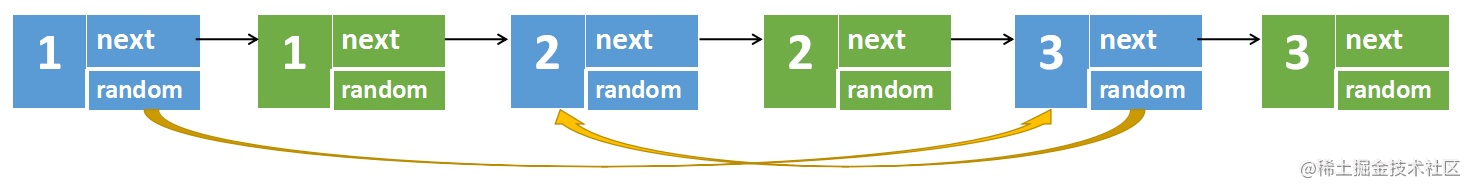2）第二步是最关键的一步，用来设置新链表的随机指针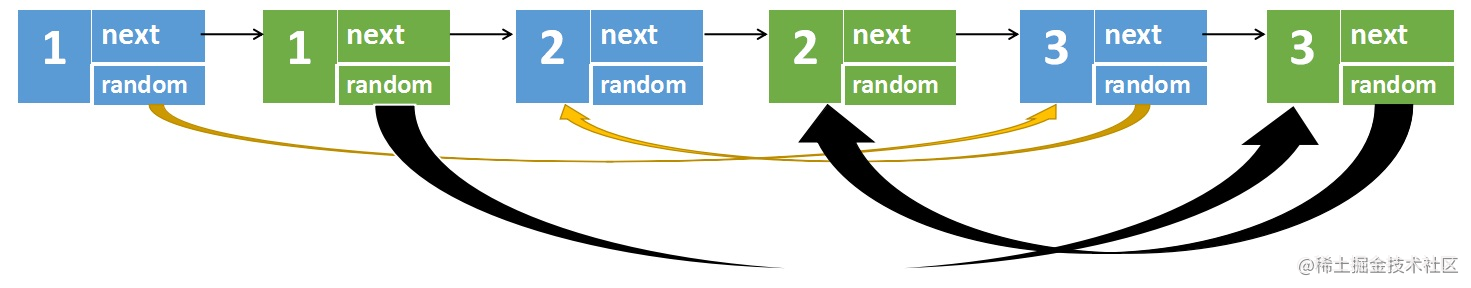• 原节点1的随机指针指向原节点3，新节点1的随机指针指向的是原节点3的next
• 原节点3的随机指针指向原节点2，新节点3的随机指针指向的是原节点2的next

3）第三步只要将两个链表分离开，再返回新链表就可以了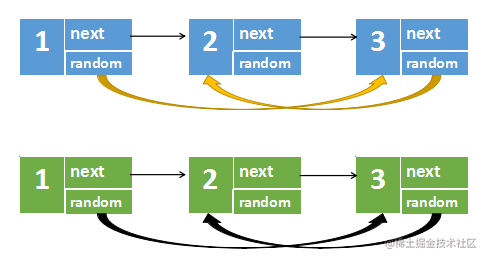``````/**
* // Definition for a Node.
* function Node(val, next, random) {
*    this.val = val;
*    this.next = next;
*    this.random = random;
* };
*/

/**
* @return {Node}
*/
return null
}

// 在每个原节点后面创建一个新节点
while(p !== null){
let newNode = new Node(p.val)
newNode.next = p.next
p.next = newNode
p = newNode.next
}
// 设置新节点的随机节点
while(p !== null){
if(p.random){
p.next.random = p.random.next
}
p = p.next.next
}
// 将两个链表分离
while(p !== null){
cur.next = p.next
cur = cur.next
p.next = cur.next
p = p.next
}
};

• 时间复杂度：O(n)，其中 n 是链表中节点的数目，我们需要遍历整个链表。
• 空间复杂度：O(n)，这里我们只需要常量的空间。

#### （20）对链表进行插入排序

1. 插入排序是迭代的，每次只移动一个元素，直到所有元素可以形成一个有序的输出列表。
2. 每次迭代中，插入排序只从输入数据中移除一个待排序的元素，找到它在序列中适当的位置，并将其插入。
3. 重复直到所有输入数据插入完为止。

``````输入: 4->2->1->3

``````输入: -1->5->3->4->0

1. 首先判断给定的链表是否为空，若为空，则不需要进行排序，直接返回。
3. 维护 last 为链表的已排序部分的最后一个节点，初始时 last = head。
4. 维护 cur 为待插入的元素，初始时 cur = head.next。
5. 比较 last 和 cur 的节点值。
• 若 last.val <= cur.val，说明 cur 应该位于 last 之后，将 last后移一位，curr 变成新的 last。
• 否则，从链表的头节点开始往后遍历链表中的节点，寻找插入 cur 的位置。令 pre 为插入 cur 的位置的前一个节点，进行如下操作，完成对 cur 的插入：
``````last.next = cur.next
cur.next = pre.next
pre.next = cur

1. 令 cur = last.next，此时 cur 为下一个待插入的元素。
2. 重复第 5 步和第 6 步，直到 cur 变成空，排序结束。
``````/**
* function ListNode(val, next) {
*     this.val = (val===undefined ? 0 : val)
*     this.next = (next===undefined ? null : next)
* }
*/
/**
* @return {ListNode}
*/
}

while(cur){
if(last.val <= cur.val){
last = last.next
}else{
while(pre.next.val <= cur.val){
pre = pre.next
}
last.next = cur.next
cur.next = pre.next
pre.next = cur
}
cur = last.next
}
};

• 时间复杂度：O(n)，对于链表而言，插入元素时只要更新相邻节点的指针即可，因此插入操作的时间复杂度是 O(1)，但是找到插入位置需要遍历链表中的节点，时间复杂度是 O(n)，因此链表插入排序的总时间复杂度仍然是 O(n)，其中 n 是链表的长度。
• 空间复杂度：O(1)，需要额外的常数大小的辅助空间。

#### （21）奇偶链表

``````输入: 1->2->3->4->5->NULL

``````输入: 2->1->3->5->6->4->7->NULL

• 应当保持奇数节点和偶数节点的相对顺序。
• 链表的第一个节点视为奇数节点，第二个节点视为偶数节点，以此类推。

• 定义a和b两个链表，分别表示奇数和偶数节点，用node来暂时保存b链表的头结点，便于后面拼接
• 使用while循环对a和b链表进行交叉遍历赋值。a和b一直指向奇数链和偶数链的最后一个节点
• 将两个链表进行拼接，并返回
``````/**
* function ListNode(val, next) {
*     this.val = (val===undefined ? 0 : val)
*     this.next = (next===undefined ? null : next)
* }
*/
/**
* @return {ListNode}
*/
}
let a = head       // 存放奇数节点
let b = head.next  // 存放偶数节点
const node = head.next   // 记录b链表的头节点
while(b !== null && b.next !== null){
a.next = b.next
a = a.next
b.next = a.next
b = b.next
}
a.next = node
};

• 时间复杂度：O(n)。其中`n`为链表的长度，我们需要遍历整个链表。
• 空间复杂度：O(1)。需要额外的常数大小的辅助空间。